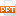# Kinh tế học - Chapter 2: Mathematical and statistical foundations

A function is a mapping or relationship between an input or set of inputs and an output We write that y, the output, is a function f of x, the input, or y = f(x) y could be a linear function of x where the relationship can be expressed on a straight line Or it could be non-linear where it would be expressed graphically as a curve If the equation is linear, we would write the relationship as y = a + bx where y and x are called variables and a and b are parameters a is the intercept and b is the slope or gradient60 trang | Chia sẻ: thuychi16 | Lượt xem: 631 | Lượt tải: 0
Bạn đang xem trước 20 trang tài liệu Kinh tế học - Chapter 2: Mathematical and statistical foundations, để xem tài liệu hoàn chỉnh bạn click vào nút DOWNLOAD ở trên